# A researcher focusing on birth weights of babies found that the mean birth weight is 3367...A researcher focusing on birth weights of babies found that the mean birth weight is 3367 grams (7 pounds, 6.8 ounces) with a standard deviation of 580 grams. Complete parts (a) and (b). a. Identify the variable Choose the correct variable below. O A. The number of births per capita OB. The accuracy of the measurements of baby birth weights OC. The weights of the babies at birth OD. The number of babies that were bom b. For samples of size 200, find the mean and standard deviation, of all possible sample mean weights. - Type an integer or a decimal. Do not round.) :- (Round to two decimal places as needed.) Click to select your answers).

Hello

A. OPTION C : The weights of babies at birth

B. For a sample size of 200,

Mean = 3367

S.d. = 580/(200^0.5)= 41.01

Thanks

#### Earn Coin

Coins can be redeemed for fabulous gifts.

Similar Homework Help Questions
• ### A researcher focusing on birth weights of babies found that the mean birth weight is 3361...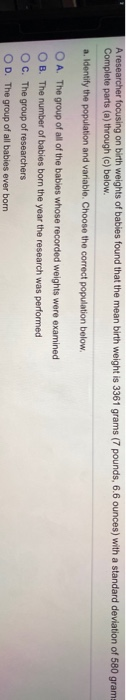A researcher focusing on birth weights of babies found that the mean birth weight is 3361 grams (7 pounds, 6.6 ounces) with a standard deviation of 580 gram Complete parts (a) through (c) below. a. Identify the population and variable. Choose the correct population below. O A. The group of all of the babies whose recorded weights were examined OB. The number of babies born the year the research was performed OC. The group of researchers OD. The group of...

• ### A scientist has read that the mean birth weight of babies bom at full term is...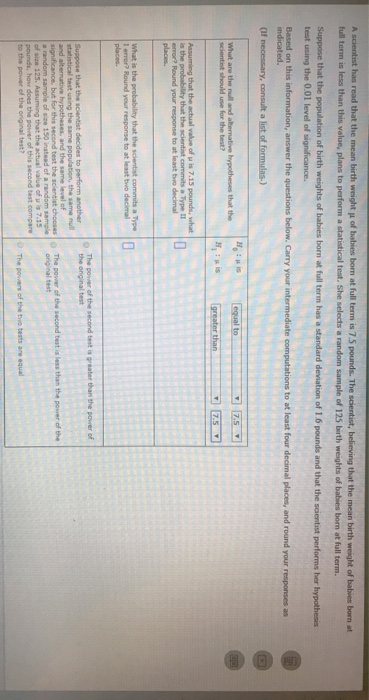A scientist has read that the mean birth weight of babies bom at full term is 75 pounds. The scientist, believing that the mean birth weight of babies bornet full term is less than this value, plans to perform a statistical test. She selects a random sample of 125 bath weights of babies bom at full term. Suppose that the population of birth weights of babies bom at full term has a standard deviation of 16 pounds and that the...

• ### A study studied the birth weights of 1,295 babies born in the United States. The mean...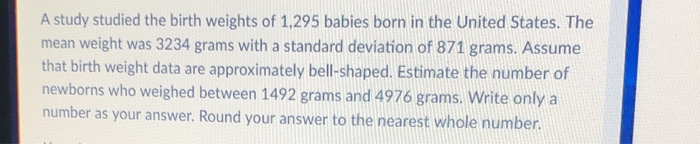A study studied the birth weights of 1,295 babies born in the United States. The mean weight was 3234 grams with a standard deviation of 871 grams. Assume that birth weight data are approximately bell-shaped. Estimate the number of newborns who weighed between 1492 grams and 4976 grams. Write only a number as your answer. Round your answer to the nearest whole number

• ### A random sample of the birth weights of 186 babies has a mean of 3103 grams...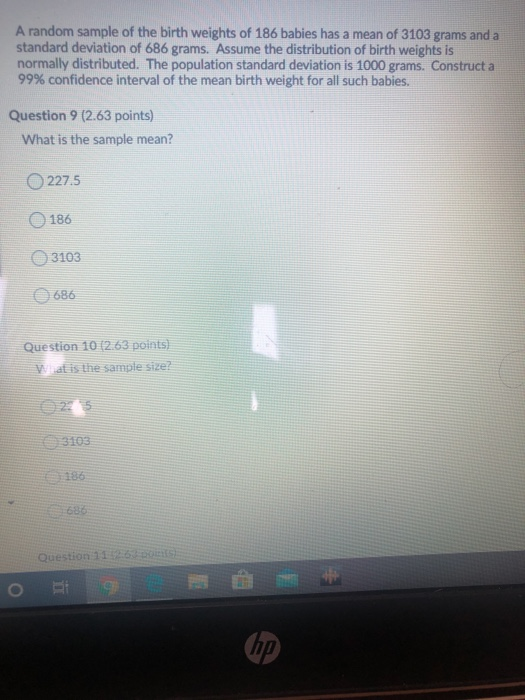A random sample of the birth weights of 186 babies has a mean of 3103 grams and a standard deviation of 686 grams. Assume the distribution of birth weights is normally distributed. The population standard deviation is 1000 grams. Construct a 99% confidence interval of the mean birth weight for all such babies. Question 9 (2.63 points) What is the sample mean? 227.5 186 3103 686 Question 10 (2.63 points) Wat is the sample size? Question 116) BE hp

• ### The birth weights for two groups of babies were compared in a study. In one group...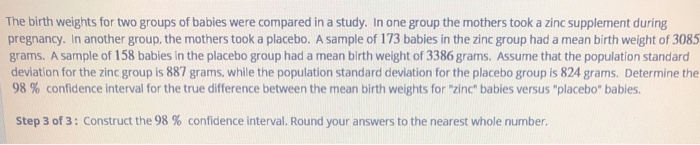The birth weights for two groups of babies were compared in a study. In one group the mothers took a zinc supplement during pregnancy. In another group, the mothers took a placebo. A sample of 173 babies in the zinc group had a mean birth weight of 3085 grams. A sample of 158 babies in the placebo group had a mean birth weight of 3386 grams. Assume that the population standard deviation for the zinc group is 887 grams, while...

• ### A study studied the birth weights of 2,932 babies born in the United States. The mean...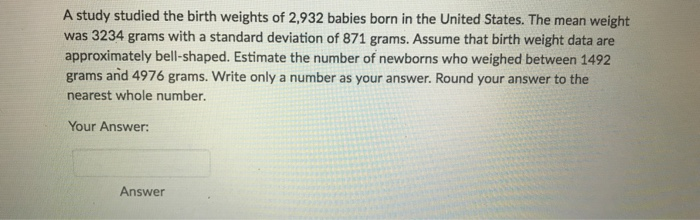A study studied the birth weights of 2,932 babies born in the United States. The mean weight was 3234 grams with a standard deviation of 871 grams. Assume that birth weight data are approximately bell-shaped. Estimate the number of newborns who weighed between 1492 grams and 4976 grams. Write only a number as your answer. Round your answer to the nearest whole number. Your Answer Answer

• ### 4 .A random sample of the birth weights of 24 babies has a mean of 3103...

4 .A random sample of the birth weights of 24 babies has a mean of 3103 grams and a standard deviation of 696 grams. Construct a 95% confidence interval estimate of the mean birth weight for all such babies. if possible include graph

• ### The birth weights for two groups of babies were compared in a study. In one group...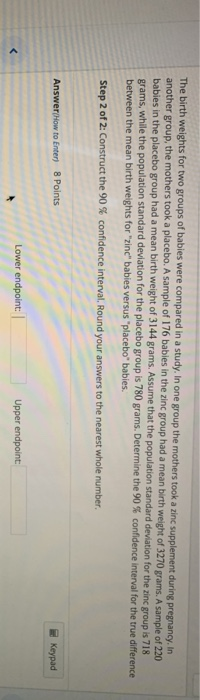The birth weights for two groups of babies were compared in a study. In one group the mothers took a zinc supplement during pregnancy. In another group, the mothers took a placebo. A sample of 176 babies in the zinc group had a mean birth weight of 3270 grams. A sample of 220 babies in the placebo group had a mean birth weight of 3144 grams. Assume that the population standard deviation for the zinc group is 718 grams, while...

• ### Suppose the birth weights of​ full-term babies are normally distributed with mean 3600 grams and standard...Suppose the birth weights of​ full-term babies are normally distributed with mean 3600 grams and standard deviation σ = 480 grams. Complete parts​ (a) through​ (c) below. Suppose the birth weights of full-term babies are normally distributed with mean 3600 grams and standard de ation σ=480 grams. Complete parts a through c) below. (a) Draw a normal curve with the parameters labeled. Choose the corect graph below O A. C. Ο D. 3120/4080 28404560 264013600 3600 3600 3120 (b) Shade...

• ### 3.4 Assess Your Understanding Applying the Concepts #5 Applying the Concepts NW 5. Birth Weights Babies...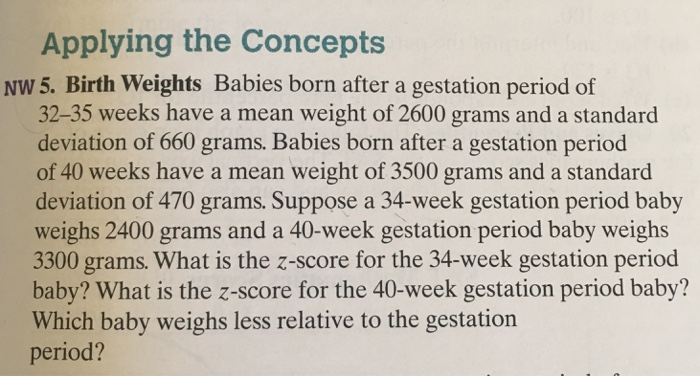3.4 Assess Your Understanding Applying the Concepts #5 Applying the Concepts NW 5. Birth Weights Babies born after a gestation period of 32-35 weeks have a mean weight of 2600 grams and a standard deviation of 660 grams. Babies born after a gestation period of 40 weeks have a mean weight of 3500 grams and a standard deviation of 470 grams. Suppose a 34-week gestation period baby weighs 2400 grams and a 40-week gestation period baby weighs 3300 grams. What...